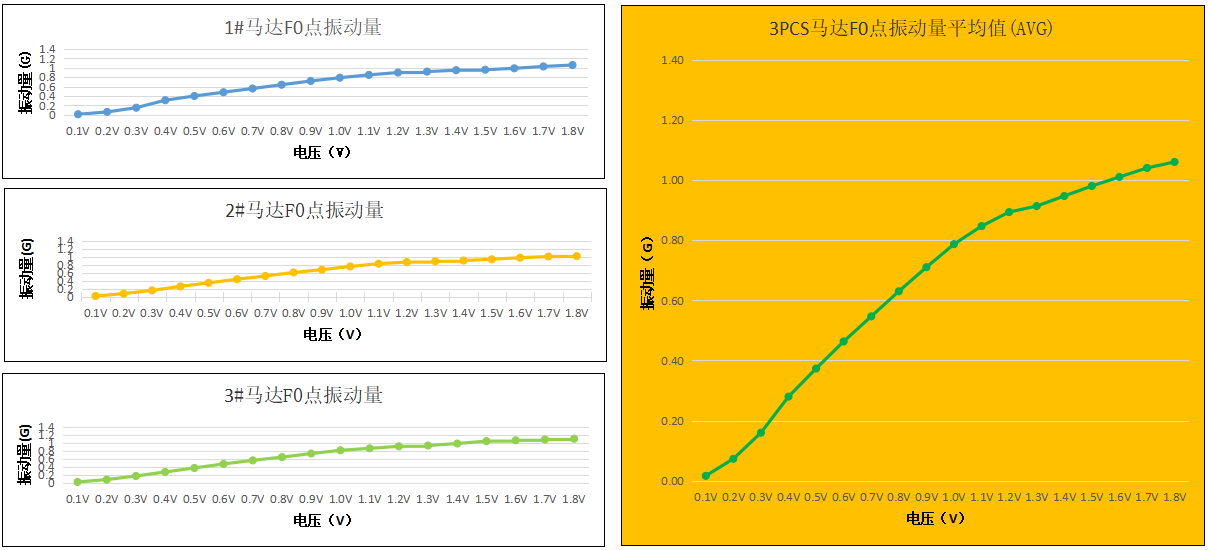# Why Use Unit G for Vibration Amplitude?

G is a unit commonly used to describe the amplitude of vibration in vibration motors and linear resonant actuators. It represents the acceleration due to gravity, which is approximately 9.8 meters per second squared (m/s²).

When we say a vibration level of 1G, it means that the vibration amplitude is equivalent to the acceleration an object experiences due to gravity. This comparison allows us to understand the intensity of the vibration and its potential impact on the current system or application.

It is important to note that G is just a way of expressing the amplitude of vibration, it can also be measured in other units such as meters per second squared (m/s²) or millimeters per second squared (mm/s²), depending on the specific requirements or standard. Nonetheless, using G as a unit provides a clear reference point and helps customers understand vibration levels in a relevant way.## What is the reason for not using displacement (mm) or force (N) as a measure of vibration amplitude?

Vibration motors are typically not used alone. They are often incorporated into larger systems along with target masses. To measure vibration amplitude, we mount the motor on a known target mass and use an accelerometer to collect the data. This gives us a clearer picture of the overall vibration characteristics of the system, which we then illustrate in a typical performance characteristics diagram.

The force exerted by the vibration motor is determined by the following equation:

$$F = m \times r \times \omega ^{2}$$

(F) represents the force, (m) represents the mass of the eccentric mass on the motor (regardless of the entire system), (r) represents the eccentricity of the eccentric mass, and (Ω) represents the frequency.

It should be noted that only the vibration force of the motor ignores the influence of the target mass. For example, a heavier object requires greater force to produce the same level of acceleration as a smaller and lighter object. So if two objects use the same motor, the heavier object will vibrate to a much smaller amplitude, although the motors produce the same force.

Another aspect of the motor is the vibration frequency:

$$f = \frac{Motor \: Speed \:(RPM)}{60}$$

The displacement caused by vibration is directly affected by the frequency of vibration. In a vibrating device, forces act cyclically on the system. For every force exerted, there is an equal and opposite force that eventually cancels it. When the frequency of vibration is higher, the time between the occurrence of opposing forces decreases.

Therefore, the system has less time to be displaced before opposing forces cancel it out. Additionally, a heavier object will have a smaller displacement than a lighter object when subjected to the same force. This is similar to the effect mentioned earlier regarding force. A heavier object requires more force to achieve the same displacement as a lighter object.

Our team can provide support and assistance with motor products. We understand that understanding, specifying, validating and integrating motor products into end applications can be complex. We have the knowledge and expertise to help reduce the risks associated with motor design, manufacturing and supply. Contact our team today to discuss your motor-related needs and find a solution that suits your specific requirements. We're here to help.

We help you avoid the pitfalls to deliver the quality and value your micro brushless motor need, on-time and on budget.